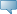# LCC

## Detailed Course Information

Spring 2012
Feb 25, 2020When the term course schedule is available the "Schedule Types" are hyperlinks. Clicking on the link will then display individual section information.

 MATH 114 - Technical Math I Introductory applied algebra for students in technical programs, with brief introduction to trigonometry. Algebraic expressions, powers, roots, ratio and proportion, variation, linear and quadratic equations, evaluating and solving formulas, systems of equations, graphing, area, volume, Pythagorean Theorem, brief introduction to right triangle trigonometry. Emphasizes problem-solving for technical applications. (F,Sp,Su) Prerequisite: (Minimum 2.0 in MATH 050 within 2 years or Math Level 4 within 2 years) and Reading Level 4 Course Note: Technical career programs allow students with Math Level 5 and Reading Level 5 to enroll directly in MATH 115, even if MATH 114 is listed in the Requirements. 4.000 Credit hours 64.000 Lecture hours 0.000 Lab hours 0.000 Other hours Levels: Credit Schedule Types: Lecture Arts and Sciences Division Math and Computer Science Dept Department Bookstore Information Restrictions: May not be enrolled in one of the following Levels:            Lifelong Learning